# SAT Math Multiple Choice Question 924: Answer and Explanation

### Test Information

Question: 924

8. If g(x + 3) = x2 + 2x + 1, then which of the following gives g(x)?

• A.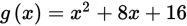• B.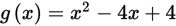• C.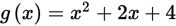• D.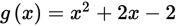Explanation:

B

Difficulty: Hard

Category: Passport to Advanced Math / Functions

Strategic Advice: The key to answering a question like this is having a conceptual understanding of function notation. Here, the input (x + 3) has already been substituted and simplified in the given function. Your job is to determine what the function would have looked like had x been the input instead.

Getting to the Answer: To keep things organized, let u = x + 3, the old input. This means x = u - 3. Substitute this into g(x + 3) and simplify: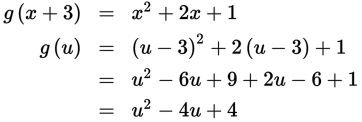This means g(u) = u2 - 4u + 4. Now, when working with function notation, you evaluate the function by substituting a given input value for the variable in the parentheses. Here, if the input value is x, then g(x) = x2 - 4x + 4, which is (B).